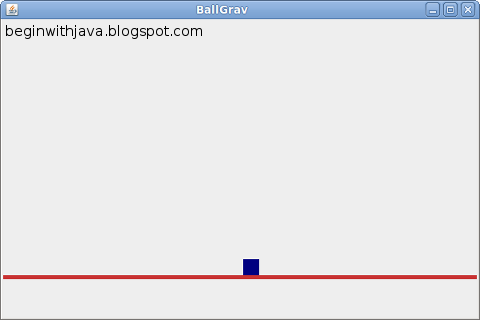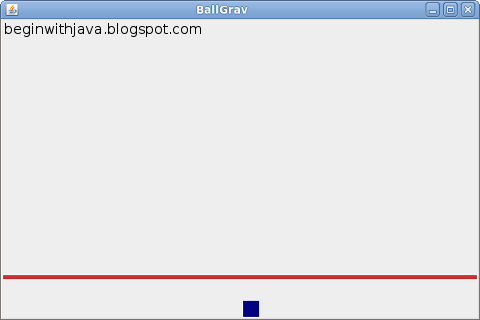## Wednesday, August 18, 2010

### Stopping Jonathan Livingston Seagull with Java

In Simple Collision Detection we used the intersects() method of two Rectangle subclasses to see if one object struck another on our playfield. It works, in the example given. But in the example given the velocity of the ball is limited.In the VGBKernel.java example, we have a fairly slow-moving ball detecting collisions with a really large object that's hard to miss.

If we have a brick that is small, and a ball that is fast, though, the ball could pass right over the brick without even realizing it should have collided with it. In other words, if we had a brick wall stretching across the screen, the ball might fly "through" it when we want it to stop or bounce. Let's say we're falling and want to detect a brick that acts as a floor. The floor goes across the screen between heights 100 and 105. The ball starts at location 75 and has a velocity of 50. Its new location would be 125. We move it there, and check for a collision as we did in VGBKernel. No collision. The ball just flew "through" the brick wall like Jonathan Livingston Seagull!

Let's make an example program called BallGrav.java to illustrate the problem. BallGrav.java is a slightly modified version of VGBKernel.java. It uses the same Brick class as we've used before, with no modifications.
`/* Based on the video game style kernel, revision 3.   by Mark Graybill, August 2010   Demonstrates collision detection problems, and solutions.*/// Import Timer and other useful stuff:import java.util.*;// Import the basic graphics classes.import java.awt.*;import javax.swing.*;import java.lang.Math;public class BallGrav extends JPanel{public Rectangle screen, bounds; // The screen area and boundary.public JFrame frame; // A JFrame to put the graphics into.public VGTimerTask vgTask; // The TimerTask that runs the game.public VGBall ball; // The game ball, a subclass of Rectangle.private Brick brick; // A brick for the ball to interact with.// Create a constructor method:  public BallGrav(){    super();    screen = new Rectangle(0, 0, 600, 400);    bounds = new Rectangle(0, 0, 600, 400); // Give some temporary values.    ball = new VGBall();    frame = new JFrame("BallGrav");    vgTask = new VGTimerTask();    brick = new Brick();}  // Create an inner TimerTask class that has access to the  // members of the BallGrav.  class VGTimerTask extends TimerTask{    public void run(){      ball.move();      frame.repaint();    }  }  // Create an inner VGBall class that has our game logic in it.  class VGBall extends Rectangle{    int xVel, yVel; // The ball's velocity.    Color ballColor; // The color of the ball.    public VGBall(){      super(300, 0, 20, 20);      xVel = 0; // Start with 0 velocity at center top of screen.      yVel = 0;      ballColor=new Color(0, 0, 128);    }    // Instance methods for VGBall    public void move(){      // Accelerate due to "gravity".      yVel+=3;      // Move the ball according to the game rules.      x+=xVel; // Move horizontally.      y+=yVel; // Move vertically, accelerating as we go.      // Detect edges and stop movement if we hit them.      if (x > (bounds.width - width)){        xVel = 0; // stop movement.        x = bounds.width -  width; // Set location to screen edge.      }      if (y > (bounds.height - height)){        yVel = 0; // stop movement.        y = bounds.height - height;      }      if (x <= 0) { xVel = 0; x = 0; }      if (y <= 0) { yVel = 0; y = 0; }      // Check for intersection with Brick,      // change color when touching.      if (intersects(brick)) {        //Stop on top of the brick if we hit it.        yVel = 0;        y = brick.y - height;      }    }    public void draw(Graphics g){    // the ball draws itself in the graphics context given.      Color gcColor = g.getColor(); // Preserve the present color.      g.setColor(ballColor); // Use the ball's color for the ball.      g.fillRect(x, y, width, height); // Draw the ball.      g.setColor(gcColor); // Restore prior color.    } // end draw()  } // end of class VGBall// Now the instance methods:  public void paintComponent(Graphics g){    // Get the drawing area bounds for game logic.    bounds = g.getClipBounds();    // Clear the drawing area.    g.clearRect(screen.x, screen.y, screen.width, screen.height);    // Draw the brick.    g.setColor(brick.getColor());    g.fillRect(brick.x, brick.y, brick.width, brick.height);    // Draw the ball.    ball.draw(g);  }  public static void main(String arg[]){    java.util.Timer vgTimer = new java.util.Timer();  // Create a Timer object    BallGrav panel = new BallGrav();         panel.frame.setDefaultCloseOperation(JFrame.EXIT_ON_CLOSE);    panel.frame.setSize(panel.screen.width, panel.screen.height);    panel.frame.setContentPane(panel);     panel.frame.setVisible(true);    // Set up the brick.    panel.brick.x = 0;    panel.brick.y = 4 * (panel.screen.height/5);    panel.brick.width = panel.screen.width;    panel.brick.height = 5;    panel.brick.brickColor = new Color(200,50,50);    // Set up a timer to do the vgTask regularly.    vgTimer.schedule(panel.vgTask, 250, 200);  }}`
This example functions properly. When the ball falls, it strikes the brick floor and stops.But let's make the ball accelerate a bit faster. Make the following change in Ball's move() method:
`      // Accelerate due to "gravity".      yVel+=5;`

Suddenly, the ball doesn't detect the floor any more, and it goes to the bottom of the screen, right through the floor:Stopping Jonathan Livingston Gameball from Going Through the Wall

What do we do to keep this from happening? Well, one way is to never let the ball move faster than it can detect collisions. Then we'd never let it move more than its own size in any direction. If it's important that it know what side it hits something on (like when we're going to bounce off it), then we may not want to let it go more than half its size in any direction in any one "move." To do this to BallGrav,java, we'd change the code as follows:
`      // Accelerate due to "gravity".      yVel+=5;      // Limit our velocity to preserve collisions.      if (yVel>height) { yVel = height; }`

Now the ball will never go so fast it never sees the floor.

But what if we want the ball to move faster?

In this case, there are a number of possible solutions. Many of them are fairly complicated, with lots of math or with lots of iteration checking for collisions at each step along the way. Complexity is something we don't want, for a lot of reasons. Here is one possible solution, that uses collision prediction to see if our ball might hit something along the way:

`      // Accelerate due to "gravity".      yVel+=5;      // Limit our velocity.      if (yVel>(height*2)) { yVel = height * 2; }      // Move the ball according to the game rules.      // Check for a collision if we're moving fast.      if (yVel>height) {        Rectangle halfway = new Rectangle(x,y+(yVel/2), width, height);        if (halfway.intersects(brick)) { yVel = yVel/2; }      }      x+=xVel; // Move horizontally.      y+=yVel; // Move vertically, accelerating as we go.`

Here, I have a velocity limit twice as high as before. This allows the ball to move along a lot faster (before, I could see the ball slow down.) Now, since the ball can move fast enough to fly through things, we first look ahead to see if there's anything in the way. We do this by seeing if there's something halfway there. If so, we cut our original speed in half before we move. Then the collision (if any) happens "naturally."

This is good enough, if the fastest we can move is twice the ball's height. If we allowed three times its height, we might check at points 1/3 and 2/3 of the way along its path.

If we don't want limits like this, we pay the price by having a more complex method to check for collisions. Here, I've limited things to movement in only one direction, up and down. If we're going side to side as well, I'd have to check for waypoints along a diagonal path. To get really precise, I'd have to do something like project lines along the corners of the ball from its current position to its intended new position and see if they intersect anything. When you get into more complex shapes than the rectangles we're using now, you get a different set of choices.

Fortunately, for video games, simple choices are almost always plenty good enough. Checking for collisions at the minimum number of points along the path is almost always good enough. This can be done by dividing the distance to be moved by the largest safe move distance, and checking that many times for collisions along the path.

Avoiding the Problem Entirely

You can also just use a different sort of movement that never has any of the objects moving more than some small number of pixels at a time--small enough that you'll never fail to detect a collision. I'll give an example in a future article, but for now I'll just describe it.

What you do is set a speed at which you move things only, say, one pixel per "move". But you only move them every so often, depending on what their speed is. The fastest objects get moved on every redraw of the screen. Slower objects only get moved on every other update, or every fifth, or something of that sort. That way an object is never moved across the screen by so many pixels at once that it misses seeing a collision with another object.

Collision Detection: Never "Easy"

Collision detection is one of the programmer's bugaboos. It's one of the places where errors are most prone to occur in code, and it can have terrible effects on a game if it doesn't work properly. The programmer's choices are to accept certain limitations or to make more complex code, or to use a technique of object movement that can either be limiting or eat up a lot of processor time.

When my students program their video game projects in class, one of our biggest problems in implementing those games is dealing with collision detection. Even though we're working in Greenfoot, which does a lot of the work for us. We still need to be aware of the limitations built into the system, and work within them.

Now it's your turn. Try reducing the brick in BallGrav.java to a small platform. Now give the ball a constant x velocity. See if you can get the ball to land on that platform reliably, with successful collision detection.